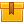# Interpreting Electrical Schematics

## Prerequisites

Knowledge of electrical schematic symbols for input, logic, and output devices.

## Description

This is the fourth lesson in the iKNOW Electrical Print Reading Library. This lesson describes the steps for interpreting the relationships among the input, logic, and output components of an electrical schematic.

## Objectives

List and define the two basic parts of an electrical schematic. Describe the layout of a typical electrical schematic. List and describe various conventions for labeling schematics. Describe the steps for interpreting schematics. Given a device in an electrical schematic, state the function of that device. Given a rung in an electrical schematic, interpret the function of that rung. Given the margin notes in an electrical schematic, determine what rungs are associated with the output device. Interpret an electrical schematic.

## ContentThis is the fourth lesson in the iKNOW™ Electrical Print Reading
Library. This lesson describes the steps for interpreting the
relationships among the input, logic, and output components of an
electrical schematic.

•Interpreting Electrical Schematics

0
0 Reviews